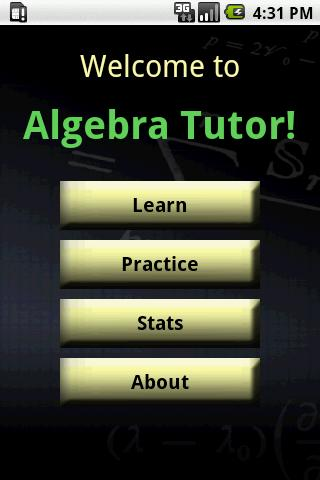# Algebra Tutor APK

Algebra Tutor APKPractice and learn Prealgebra and Algebra topics!
See your stats for every problem type (saved across app runs).
Work out problems without needing to take notes.
Get math help wherever you are.
35 practice concepts
– Find the Greatest Common Denominator
– Fraction Division
– Fraction Multiplication
– Fraction Subtraction
– Reduce Fraction to Lowest Terms
– Distributed Linear
– One Step Linear Equations Using Integers
– Two Step Linear Equations Using Integers
– Multiplying Monomials by Polynomials
– Naming Polynomials by Degree
– Naming Polynomials by Degree and Terms
– Naming Polynomials by Number of Terms
– Single Degree Simplifiable Polynomial
– Factorable Quadratic in Factored Form
– Factorable Quadratic in Standard Form
– Convert from Decimal to Scientific Notaiton
– Convert from Scientific Notation to Decimal
– English to Variable Expression
– Evaluating Single Variable Linear Expressions
– Finding Prime Numbers
– Prime or Composite?
– Classifying Rational Numbers
– Convert Expanded Form To Exponent
– Convert Exponent to Expanded Form
– Convert Exponent to Words
– Exponentiating Exponents
– Write Base and Exponent
– Expressing Fraction in Higher Terms
– Find Value to Make Fractions Equivalent
– Finding the Least Common Multiple
– Find the Reciprocal
– Identifying Numerator and Denominator
– Order of Operations with Integers
– Order of Operations with Whole Numbers### 1 Comment

• Azim says:

apps.apk

It’s fine.

This site uses Akismet to reduce spam. Learn how your comment data is processed.